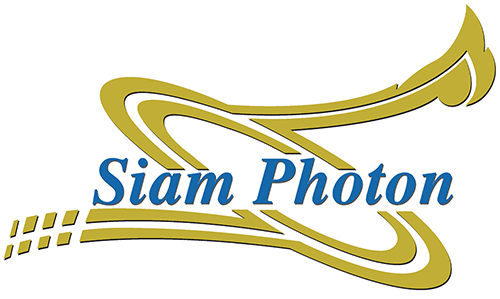Major parameters

- Function : Transport electrons from Linac to Booster Synchrotron : Energy analyzer

- Total length = 10.85   m

- Characteristic beam from LINAC to LBT

- Beam energy 40 MeV

- Beam current 30 mA

Magnet parameters

Bending magnet

- Bending radius ( ρ) = 0.5 m

- Deflection angle ( α) = 50.5 ° ( 0.8813913 rad)

- Path Length (l) = 0.4406956 m

- Max magnetic Field (B) = 0.2668 T

- Maximum current = 15.0 A

- Magnet type : C-type

- Number of quadrupole magnets = 11

- Mechanical core length = 0.1 m

- Pole radius = 86 mm

- Maximum current = 8.0 A

Steering magnet

- Number of steering magnets = 5

- Maximum current = 9.0 A

Synchrotron Light Research Institute,111 University Avenue, Muang District, Nakhon Ratchasima 30000, THAILAND
Postal address : P.O. Box 93, Muang, Nakhon Ratchasima 30000, THAILAND

Tel.: 66 44 217 040 Fax: 66 44 217 047 Email: siampl@slri.or.th